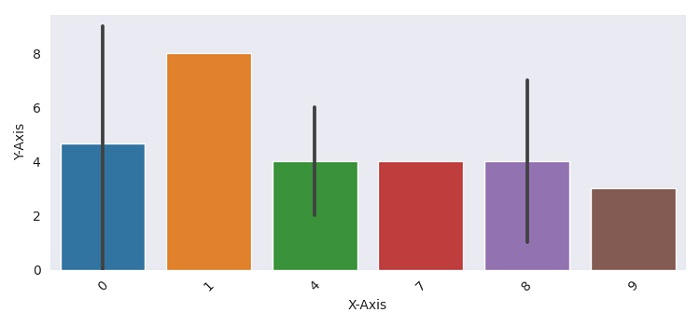# Best way to display Seaborn/Matplotlib plots with a dark iPython Notebook profile

To display a Seaborn/Matplolib plot with a dark background, we can use "dark" in set_style() method that gives an aesthetic style to the plots.

## Steps

• Set the figure size and adjust the padding between and around the subplots.

• Use "dark" in set_style() method that sets the aesthetic style.

• Create a Pandas dataframe with two columns.

• Show point estimates and confidence intervals with bars, using bar plot() method.

• Rotate xticks by 45 degrees.

• To display the figure, use show() method.

## Example

import pandas
import matplotlib.pylab as plt
import seaborn as sns
import numpy as np

plt.rcParams["figure.figsize"] = [7.50, 3.50]
plt.rcParams["figure.autolayout"] = True

sns.set_style("dark")

df = pandas.DataFrame({"X-Axis": [np.random.randint(10) for i in range(10)], "Y-Axis": [i for i in range(10)]})

bar_plot = sns.barplot(x='X-Axis', y='Y-Axis', data=df)
plt.xticks(rotation=45)

plt.show()

## Output